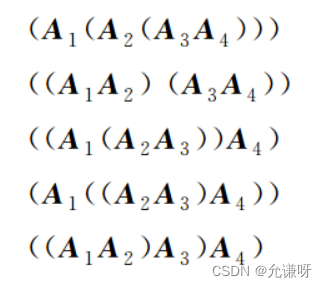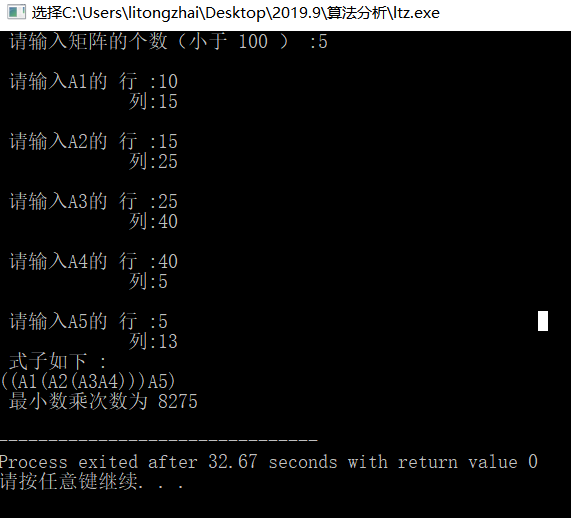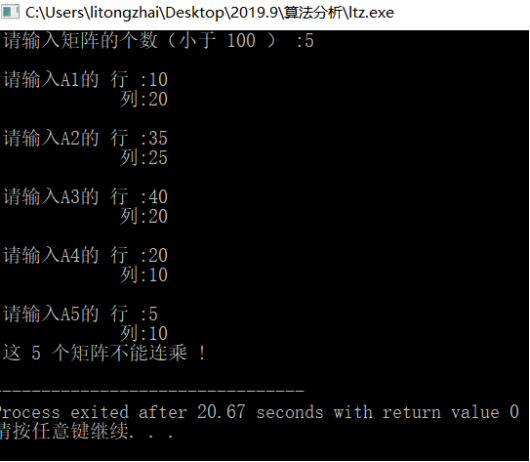• 矩阵连乘
2022-05-12 09:05:37

## 1.认真审阅题目，明确题目的已知条件和求解的目标；

给定n个矩阵{A1,A2,A3……，An},其中Ai与Ai+1（i = 1,2 ,3,4……n-1）是可乘的，加括号的方法表示矩阵连乘的次序，不同加括号的方法所对应的计算次序是不同的。

## 2.问题建模

【例4-2】三个矩阵 A1 A2 A3 连乘，用加括号的方法表示其计算次序。

3个矩阵相乘，其加括号的方法一共有两种，具体如下：

【例4-3】4个矩阵连乘，用加括号的方法表示其计算次序。

4个矩阵连乘，其加括号的方法共有5种，具体如下：不同加括号的方法所对应的计算量也是不同的，甚至差别很大。由于在矩阵相乘的过程中，仅涉及加法和乘法两种基本运算，乘法耗时远远大于加法耗时，故采用矩阵连乘所需乘法的次数来对不同计算次序的计算量进行衡量。

【例4-4】三个矩阵 A1 ,A2 ,A3 的行列分别为10×100、100×5、5×50，求例4-2中的两种加括号方法所需要乘法的次数。

两种加括号方法所需要乘法的次数分别为

那么，矩阵连乘问题就是对于给定n个连乘的矩阵，找出一种加括号的方法，使得矩阵连乘的计算量最小。

容易想到的解决方法是穷举法，即对n个矩阵连乘的每一种加括号方法进行乘法次数的统计，从中找出最小的计算量所对应的加括号方法。这种方法的复杂性取决于加括号的方法的种数。对于n个矩阵连乘，其加括号的方法有多少种呢？

考查矩阵连乘，不管哪种加括号的方法，最终都归结为两部分结果矩阵相乘，这两部分从n个连乘矩阵中的哪个矩阵处分开呢？设可能从A k 和A k+1 处将n个矩阵分成两部分，其中k=1,2,…,n-1。令P(n)代表n个矩阵连乘不同的计算次序，即不同加括号的方式，则n个矩阵连乘加括号的方式可通过两步操作来实现：①分别完成对两部分加括号；②对所得的结果加括号。由此

解此递推方程可得P(n)实际上是Catalan数，即P(n)=C(n-1)，其中 。故穷举法的复杂性非常高，是n的指数级函数，显然，该方法不可行。

## 3.算法设计；

采用自低向上的方法求解最优质的具体的求解步骤设计如下：
步骤1：确定合适的数据结构，采用二维数组m来存放各个子问题的最优值，二维数组来存放来存放各个子问题的最优策略，（如果s[i][j]=k，则最优加括号的 方法为(Ai……Ak）(Ak+1……，Aj)，一维数组P
步骤2:初始化。令m[i][j] = 0 ， s[i][j] = 0,其中 i = 1, 2, ……
步骤3：循环阶段
步骤3-1：按照递归关系式计算两个矩阵AiAi+1,相乘时的最优值，并将其存入m[i][i+1],同时将最优决策计入s[i][i+1]，i = 1, 2, ……
步骤3-2：按照递归关系式计算3个矩阵AiAi+1Ai+2相乘时的最优值并将其存入m[i][i+2]，同时最优决策计入s[i+2],i = 1, 2, ……, n – 2
以此类推
步骤3-(n-1):按照递归关系式计算n个矩阵A1，A2，……An相乘时的最优质的并将其存入m[n]，同时将最优决策计入s[n]
至此，m[n]即为原问题的最优值
步骤4：根据二维数组s记录的最优决策信息来构造最优解
步骤4-1：递归构造A1,……，As[n]的最优解，直到包含一个矩阵结束
步骤4-2：递归构造As[n]+1……An的最优解，直到包含一个矩阵结束
步骤4-3：将步骤4-1和步骤4-2递归的结果加括号

## 4.编码实现；


cpp
#include <iostream>
using namespace std;
void MatrixChain(int *p, int n, int  * * m, int * * s)//用于求最优值
{
//初始化
for(int i = 1; i <= n; i ++){
m[i][i] = 0;
s[i][i] = 0;
}
for(int r = 2; r <= n; r++){//不同规模的子问题
for(int i = 1; i <= n - r + 1; i++){//每一个规模为r的矩阵连乘序列的首矩阵Ai
int j = i + r - 1;//每个规模为r的矩阵连乘序列的尾矩阵为Aj
m[i][j] = m[i+1][j] + p[i-1] * p[i]*p[j];//决策为k=i的乘法次数
s[i][j] = i;
for(int k = i + 1; k < j; k++){
int  t = m[i][k] + m[k+1][j] + p[i-1]*p[k]*p[j];
if(t < m[i][j]){
m[i][j] = t;
s[i][j] = k;
}
}
}

}
}
void Traceback(int i, int j, int ** s){
//s[i][j]记录了断开的位置，即计算A[i:j]的加括号方式为(A[i:s[i][j]]) * A [s[i][j] + 1: j])
if(i == j) return;
Traceback(i, s[i][j], s);//递归打印A[i:s[i][j]的加括号方式
Traceback(s[i][j] + 1, j, s);//递归打印A[s[i][j]+1:j ]的加括号的方式
cout << "A" << "[" << i << ":" << s[i][j] << "]" << "乘以" << "A""[" << (s[i][j] + 1) << ":" << j << "]"<< endl;
}
int main(){

return 0;
}


## 5.算法分析；

显然，语句int t = m[i][k] + m[k+1][j] + p [i-1= * p[k]*p[j]为算法MatriChain的基本语句，最坏的情况下该语句的执行次数为O（n的三次方）故散发的最坏时间复杂度为O（n的三次方）
9.总结。c++ 算法
更多相关内容
• 本文实例讲述了动态规划之矩阵连乘问题Python实现方法。分享给大家供大家参考，具体如下： 给定n个矩阵｛A1,A2,…,An｝，其中Ai与Ai+1是可乘的，i=1,2 ,…,n-1。如何确定计算矩阵连乘积的计算次序，使得依此次序计算...
• 主要介绍了C语言矩阵连乘 (动态规划)详解的相关资料,需要的朋友可以参考下
• 【问题描述】使用动态规划算法解矩阵连乘问题，具体来说就是，依据其递归式自底向上的方式进行计算，在计算过程中，保存已子问题答案，每个子问题只解决一次，在后面计算需要时只要简单查一下得到其结果，从而避免...python
• 主要介绍了Java矩阵连乘问题(动态规划)算法,结合实例形式分析了java实现矩阵连乘的算法原理与相关实现技巧,需要的朋友可以参考下
• 实现计算多个矩阵连乘的最终结果并且对时间复杂度进行了优化
• 用C++写的完整的矩阵连乘算法的实现，完整的工程。最经典的动态规划问题的算法实现。
• 算法设计与分析实验报告，附已通过源码，供学习参考，共勉♪ 目录摘要如下： 1.问题描述 2.实验目的 3.实验原理 4.实验设计 ...（包括输入格式、算法、输出格式） ...（除了截图外，实验结果还用图表进行了分析） ...
• c/c++语言解决矩阵连乘文题，几个矩阵相乘求最佳结合顺序
• 你的任务是要确定矩阵连乘的运算次序，使计算这n个矩阵的连乘积A1A2…An时总的元素乘法次数达到最少。 例如：3个矩阵A1，A2，A3，阶分别为10×100、100×5、5×50，计算连乘积A1A2A3时按（A1A2）A3所需的元素乘法...
• 南邮动态规划法实验之矩阵连乘问题，使用了备忘录法以及动态规划法实现求解，代码注释详尽
• 动态规划，矩阵连乘最优值，对于矩阵连乘中矩阵发排序，应用动态规划计算C++
• 动态规划解决矩阵连乘问题，随机产生矩阵序列，输出形如((A1(A2A3))(A4A5))的结果。 代码： #encoding: utf-8 =begin author: xu jin, 4100213 date: Oct 28, 2012 MatrixChain to find an optimum order by using ...
• 有大神能不能看一下这个矩阵连乘给我完善下，我太笨了，C++
• 动态规划算法中矩阵连乘的实现代码，主要是用C语言编写；
• ## 矩阵连乘

千次阅读 2019-12-17 17:09:11
确定计算矩阵连乘积的计算次序，使得依此次序计算矩阵连乘积需要的数乘次数最少。输入数据为矩阵个数和每个矩阵规模，输出结果为计算矩阵连乘积的计算次序和最少数乘次数。这种计算次序可以用加括号的方式来确定。...

给定n个矩阵：A1,A2,...,An，其中Ai与Ai+1是可乘的，i=1，2...，n-1。确定计算矩阵连乘积的计算次序，使得依此次序计算矩阵连乘积需要的数乘次数最少。输入数据为矩阵个数和每个矩阵规模，输出结果为计算矩阵连乘积的计算次序和最少数乘次数。这种计算次序可以用加括号的方式来确定。若一个矩阵连乘积的计算次序完全确定，也就是说该连乘积已完全加括号，则可以依此次序反复调用 2 个矩阵相乘的标准算法计算出矩阵连乘积。完全加括号的矩阵连乘积可递归地定义为：

（ 1 ）单个矩阵是完全加括号的；

（ 2 ）矩阵连乘积 A 是完全加括号的，则 A 可表示为 2 个完全加括号的矩阵连乘积 B 和 C 的乘积并加括号，即 A= （ BC ）。

分析：

1. 矩阵连乘的条件：第一个矩阵的列等于第二个矩阵的行，此时两个矩阵是可乘的；

2. 多个矩阵连乘的结果矩阵，其行等于第一个矩阵的行和其列等于最后一个矩阵的列

3.两个矩阵相乘的计算量：

例如：A（4*2），B（2*5 可知总执行次数为：4×2×5=40

所以矩阵A m*n和B n*k的乘法运算次数为：m*n*k；

1. 子问题的拆分，在（AiAi+1……Aj）中，先选择一个矩阵Ak，这样的话就将一个大矩阵拆分为两个小矩阵;假设在第k位置上找到最优解，则问题变成了两个子问题：（AiAi+1……Ak），（Ak+1……Aj）用m[i][j]表示矩阵连乘的最优值，那么两个子问题对应的最优值变成m[i][k],m[k+1][j];
2. 矩阵连乘最优值递归式：
#include <stdio.h>
#define N 100// 定义最大连乘的矩阵个数为 100 个
void matrixChain (int p[],int m[N+1][N+1],int s[N+1][N+1])
/* 用 m[i][j] 二维数组来存储 Ai*......Aj 的最小数乘次数，用 s[i][j] 来存储使 Ai......Aj 获得最小数乘次数对应的断开位置 k ，需要注意的是此处的 N+1 非常关键，虽然只用到的行列下标只从 1 到 N 但是下标 0 对应的元素默认也属于该数组，所以数组的长度就应该为 N+1*/
{
int n=N;// 定义 m,s 数组的都是 n*n 的，不用行列下标为 0 的元素，但包括在该数组中
for (int i=1;i<=n;i++)
m[i][i]=0; /* 将矩阵 m 的对角线位置上元素全部置 0 ，此时应是 r=1 的情况，表示先计算第一层对角线上个元素的值 */
for (int r=2;r<=n;r++)//r 表示斜对角线的层数，从 2 取到 n
{
for (int i=1;i<=n-r+1;i++)//i 表示计算第 r 层斜对角线上第 i 行元素的值
{
int j=i+r-1;//j 表示当斜对角线层数为 r ，行下标为 i 时的列下标
m[i][j]=m[i+1][j]+p[i-1]*p[i]*p[j];// 计算当断开位置为 i 时对应的数乘次数
s[i][j]=i;// 断开位置为 i
for (int k=i+1;k<j;k++)
{
int t=m[i][k]+m[k+1][j]+p[i-1]*p[k]*p[j];/* 计算当断开位置 k 为从 i 到 j （不包括 i 和 j ）的所有取值对应的 (Ai*......*Ak)*(Ak+1*.......Aj) 的数乘次数 */
if (t<m[i][j])
{
m[i][j]=t;// 将 Ai*......Aj 的最小数乘次数存入 m[i][j]
s[i][j]=k;// 将对应的断开位置 k 存入 s[i][j]
}
}
}
}
}
void traceback (int i,int j,int s[][N+1])// 用递归来实现输出得到最小数乘次数的表达式
{
if (i==j)
{
printf ("A%d",i);
}
else
{
printf ("(");
traceback (i,s[i][j],s);
traceback(s[i][j]+1,j,s);
printf (")");
}
}
int main ()
{
int n;// 用来存储矩阵的个数
int Q[2*N];/* 用 Q 数组来存储最原始的输入（各矩阵的行和列），主要目的是为了检验这 N 个矩阵是否满足连乘的条件 */
int p[N+1],flag=1;/* 用 p[i-1],p[i] 数组来存储 A 的阶数， flag 用来判断这 N 个矩阵是否满足连乘 */
int m[N+1][N+1];//  用 m[i][j] 二维数组来存储 Ai*......Aj 的最小数乘次数
int s[N+1][N+1];//  用 s[i][j] 来存储使 Ai......Aj 获得最小数乘次数对应的断开位置 k
printf (" 请输入矩阵的个数（小于 100 ） :");
scanf ("%d",&n);
for (int i=0;i<=2*n-1;i++)// 各矩阵的阶数的输入先存入数组 Q 中接受检验
{
if (i%2==0)
{
printf ("\n");
printf (" 请输入A%d的 行 :",(i/2)+1);
}
else
{
printf ("             列:");
}
scanf ("%d",&Q[i]);
}
for (int i=1;i<=2*n-2;i++)// 矩阵连乘条件的检验
{
if (i%2!=0&&Q[i]!=Q[i+1])
{
flag=0;
break;
}
}
for (int j=1;j<=n-1;j++)
{
p[j]=Q[2*j];
}
if (flag!=0)
{
p=Q;
p[n]=Q[2*n-1];
matrixChain (p,m,s);
printf (" 式子如下 :\n");
traceback(1,n,s);
printf ("\n");
printf (" 最小数乘次数为 %d\n",m[n]);
}
else
{
printf (" 这 %d 个矩阵不能连乘 !\n",n);
}
}`

• 实验结果与分析

（1）实验结果

1.输入正确结果的情况1. 输入有误的情况：
2.（2）分析与收获

经过这个对这个程序的了解和深入研究，渐渐理解了动态规划的基本思想。

展开全文• 第3章 动态规划;算法总体思想;但是经分解得到的子问题往往不是互相独立的不同子问题的数目常常...3.1 矩阵连乘问题;完全加括号的矩阵连乘积;完全加括号的矩阵连乘积;设有四个矩阵 它们的维数分别是;3.1 矩阵连乘问题;
• 要求计算出这n个矩阵连乘积A1A2…An最少需要多少次乘法。 输入 输入数据的第一行是一个整树n（0 ≤ 10），表示矩阵的个数。 接下来的n行每行两个整数p,q( 0 ,q )，分别表示一个矩阵的行数和列数。 输出 输出...
• 给定n个矩阵｛A1,A2,…,An｝，其中，Ai与Ai+1是可乘的，(i=1,2 ,…,n-1)。用加括号的方法表示矩阵连乘的次序，不同的计算次序计算量（乘法次数）是不同的，找出一种加括号的方法，使得矩阵连乘的次数最小
• 矩阵连乘，动态规划，直接递归，备忘录方法的比较。算法...